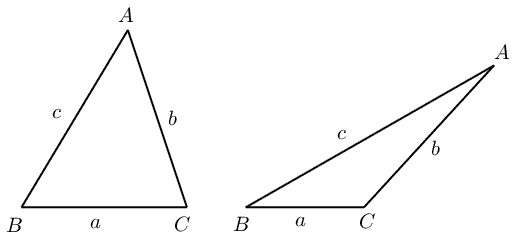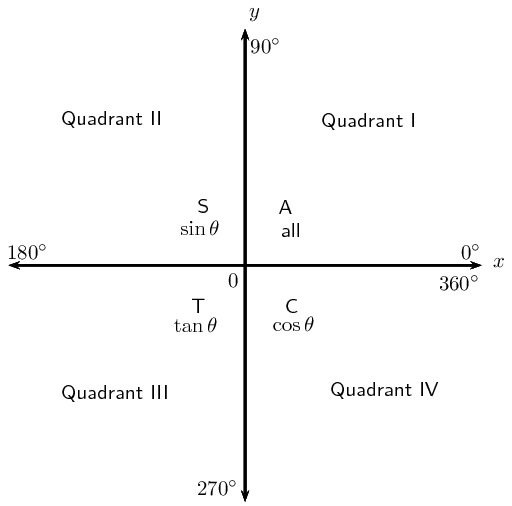Home Practice
For learners and parents For teachers and schools
Textbooks
Full catalogue
Pricing SupportLog in

We think you are located in United States. Is this correct?

# 6.6 Summary

## 6.6 Summary (EMBHT)

 square identity quotient identity $$\cos^2\theta + \sin^2\theta = 1$$ $$\tan\theta = \dfrac{\sin\theta}{\cos\theta}$$negative angles periodicity identities co-function identities $$\sin (-\theta) = - \sin \theta$$ $$\sin (\theta \pm \text{360}\text{°}) = \sin \theta$$ $$\sin (\text{90}\text{°} - \theta) = \cos \theta$$ $$\cos (-\theta) = \cos \theta$$ $$\cos (\theta \pm \text{360}\text{°}) = \cos \theta$$ $$\cos (\text{90}\text{°} - \theta) = \sin \theta$$
 sine rule area rule cosine rule $$\frac{\sin A}{a} = \frac{\sin B}{b} = \frac{\sin C}{c}$$ area $$\triangle ABC = \frac{1}{2} bc \sin A$$ $$a^2 = b^2 + c^2 - 2 bc \cos A$$ $$\frac{a}{\sin A} = \frac{b}{\sin B} = \frac{c}{\sin C}$$ area $$\triangle ABC = \frac{1}{2} ac \sin B$$ $$b^2 = a^2 + c^2 - 2 ac \cos B$$ area $$\triangle ABC = \frac{1}{2} ab \sin C$$ $$c^2 = a^2 + b^2 - 2 ab \cos C$$General solution:

1. \begin{align*} \text{If } \sin \theta &= x \\ \theta &= \sin^{-1}x + k \cdot \text{360}\text{°} \\ \text{or } \theta &= \left( \text{180}\text{°} - \sin^{-1}x \right) + k \cdot \text{360}\text{°} \end{align*}
2. \begin{align*} \text{If } \cos \theta &= x \\ \theta &= \cos^{-1}x + k \cdot \text{360}\text{°} \\ \text{or } \theta &= \left( \text{360}\text{°} - \cos^{-1}x \right) + k \cdot \text{360}\text{°} \end{align*}
3. \begin{align*} \text{If } \tan \theta &= x \\ \theta &= \tan^{-1}x + k \cdot \text{180}\text{°} \end{align*}

for $$k \in \mathbb{Z}$$.

How to determine which rule to use:

• Area rule:

• no perpendicular height is given
• Sine rule:

• no right angle is given
• two sides and an angle are given (not the included angle)
• two angles and a side are given
• Cosine rule:

• no right angle is given
• two sides and the included angle angle are given
• three sides are given
temp text